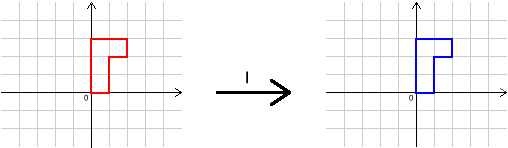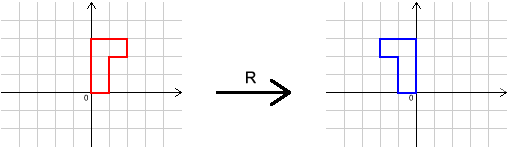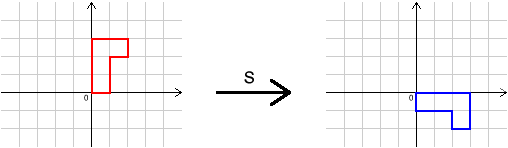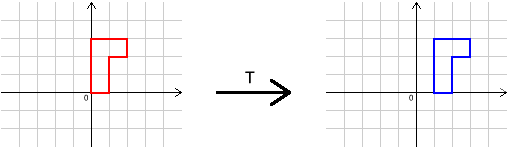#### You may also like### Chess

What would be the smallest number of moves needed to move a Knight from a chess set from one corner to the opposite corner of a 99 by 99 square board?### 2001 Spatial Oddity

With one cut a piece of card 16 cm by 9 cm can be made into two pieces which can be rearranged to form a square 12 cm by 12 cm. Explain how this can be done.### Screwed-up

A cylindrical helix is just a spiral on a cylinder, like an ordinary spring or the thread on a bolt. If I turn a left-handed helix over (top to bottom) does it become a right handed helix?

# Combining Transformations

##### Age 11 to 14 Challenge Level:

In this problem, we shall use four transformations, $I$, $R$, $S$ and $T$. Their effects are shown below.We write $R^{-1}$ for the transformation that undoes'' $R$ (the inverse of $R$), and $R S$ for "do $R$, then $S$".
We can write $T$ followed by $T$ as $T T$ or $T^2$, and $T$ followed by $T$ followed by $T$ as $T T T$ or $T^3$ and so on.
Similarly, we can write $S^{-1}S^{-1}$ as $S^{-2}$ and so on.

Try to find simpler ways to write:

$R^2$, $R^3$, $R^4$, $\dots$

$S^2$, $S^3$, $S^4$, $\dots$

$T^2$, $T^3$, $T^4$, $\dots$.

What do you notice?
Can you find a simpler way to write $R^{2006}$ and $S^{2006}$?
Can you describe $T^{2006}$?
Let's think about the order in which we carry out transformations:
What happens if you do $R S$? Do you think that $S R$ will be the same? Try it and see.
Is $T^2R$ the same as $R T^2$?
Is $(R T)S$ the same as $S(R T)$?
Try this with some other transformations.

Does changing the order always/sometimes/never produce the same transformation?

Now let's think about how to undo $R S$. What combination of $I$, $R$, $S$, $T$ and their inverses might work? Try it and see: does it work? If not, why not? Can you find a combination of transformations that does work?
How can you undo transformations like $S T$, $T R$ and $R S^2$?

This problem is the middle one of three related problems.
The first problem is Decoding Transformations and the follow-up problem is Simplifying Transformations .